##Updated: 10/13/2019

By using extended interpolation, natural motion can be expressed without texture shrinkage, such as with swaying objects.

## What is Extended Interpolation?

Extended interpolation is a function that interpolates between keyforms using curves instead of lines.
Normally, the interpolation between the keyforms of the parameters is linear.

If you rotate a warp deformer or ArtMesh directly without using the rotation deformer, the trajectory is interpolated in a straight line as shown in the figure below, so the middle point moves as if it were decreased in size.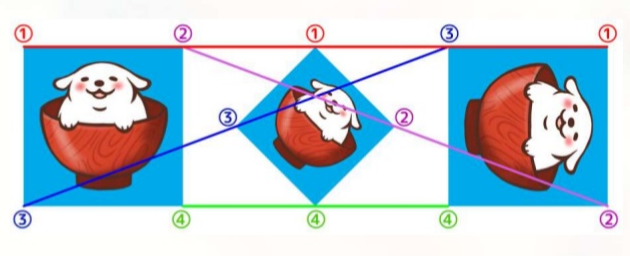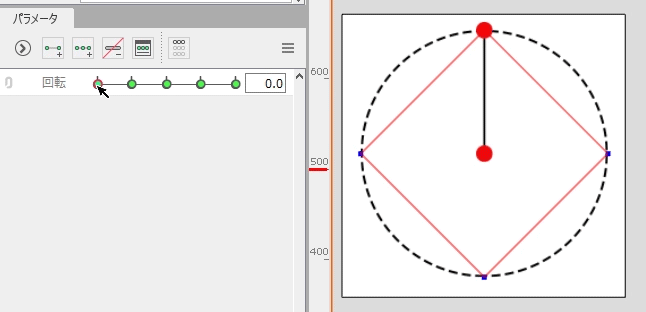By setting up extended interpolation, the interpolation calculation is performed at the timing when the parameter is manipulated, and the movement will be in the form of a circular or curved trajectory.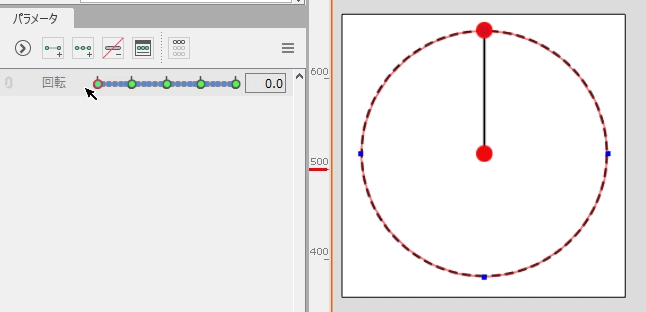## How to Set Up Extended Interpolation

1. Select the object for which you want to set SNS interpolation.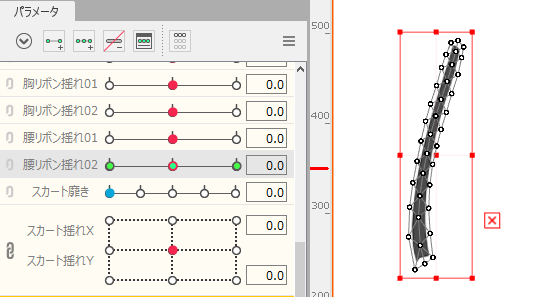2. From the [Parameter palette], click [Palette menu] -> [Extended Interpolation].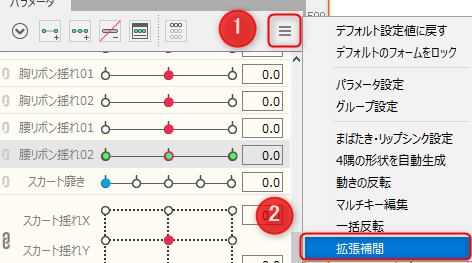3. The [Extended Interpolation] dialog box appears.
Select either [Ellipse Interpolation] or [SNS Interpolation] from [Interpolation method].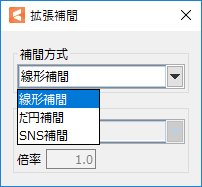Extended interpolation has been set for the parameter.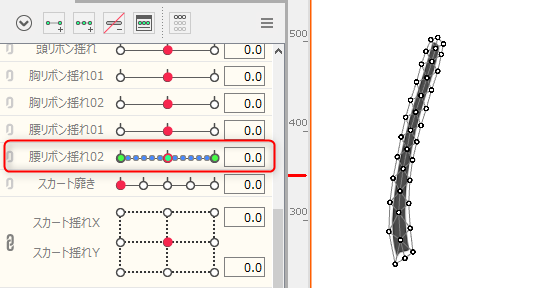## Extended Interpolation Type

Cubism offers two types of calculation methods.
See “Interpolation Type” for the characteristics and settings of each method.

### Ellipse Interpolation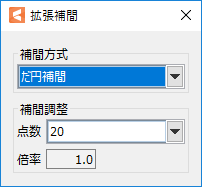The key is automatically generated on the orbit and replaces linear interpolation.
The scale and the number of key points can be adjusted, and the movement follows a circular orbit.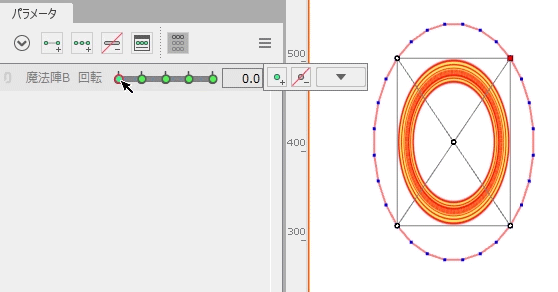### SNS Interpolation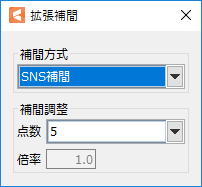Only the number of points on the orbit can be adjusted.
It can be easily used in areas where no specific trajectory is required, for example.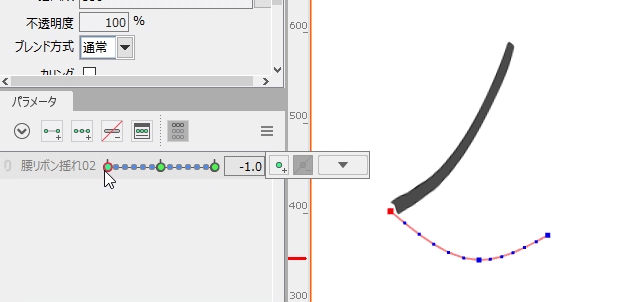## Precautions for Setup

### During parameter operation

Extended interpolation performs interpolation calculation at the timing when the parameters are manipulated, automatically generates keys on the orbit, and emulates linear interpolation at runtime.
(Interpolation calculations at runtime can affect performance.)

The more extension keys (points), the smoother the trajectory, but the drawing load in the Editor becomes heavier because the number of points is multiplied when the two parameters are multiplied together.
Although the operation is lighter when the object is deselected, it is recommended that the extension key be less than 10 points when multiplying two parameters.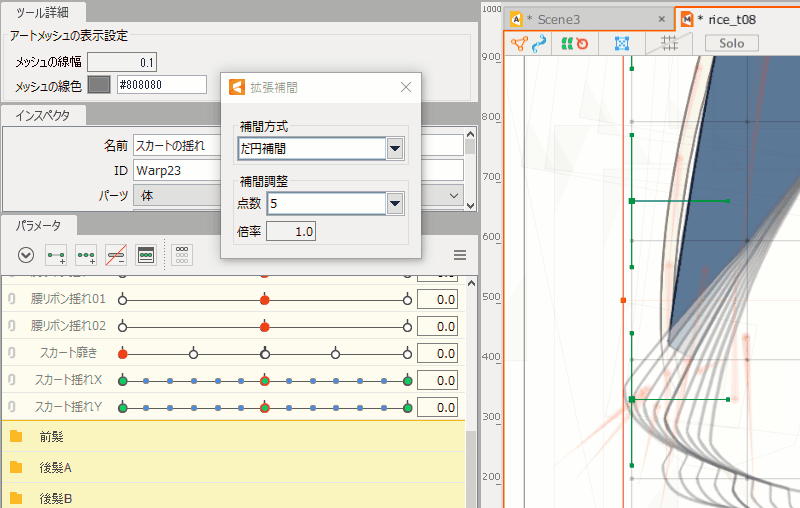###### Precautions

When the number of extended interpolation points is 20 (maximum), the key-to-key spacing should be at least 0.03, since anomalies may occur in files with key-to-key spacing less than 0.03.

### When handling extended interpolation in the SDK

Extended Interpolation is a function that curvilinearly interpolates the linear interpolation of a parameter.
In fact, it automatically generates a specified number of keyforms and keyforms for extended interpolation between the keyforms.

Therefore, when extended interpolation is set on an object with multiple parameters, the size of the model file increases proportionally because the keyforms added by extended interpolation are multiplied with each other.
Please note that when dealing with extended interpolation in the SDK, there is little effect on performance during execution, but there is a slowdown when loading the model.